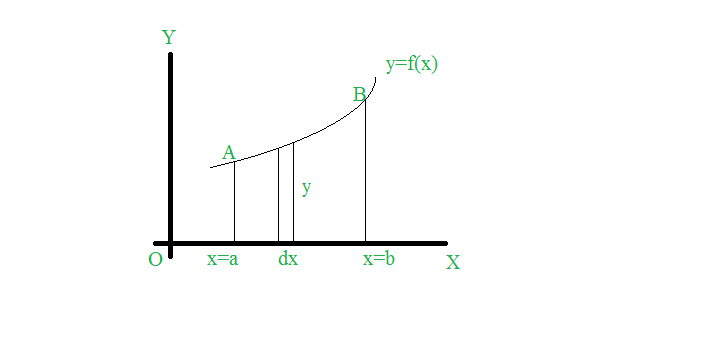Open in App
Not now

# Mathematics | Area of the surface of solid of revolution

• Difficulty Level : Medium
• Last Updated : 10 May, 2020

Consider a plane y=f(x) in the x-y plane between ordinates x=a and x=b. If a certain portion of this curve is revolved about an axis, a solid of revolution is generated.We can calculate the area of this revolution in various ways such as:

1. Cartesian Form:

• Area of solid formed by revolving the arc of curve about x-axis is-• Area of revolution by revolving the curve about y axis is-2. Parametric Form:3. Polar Form: r=f(θ)

• About the x-axis: initial lineHere replace r by f(θ)Here replace r by f(θ)

4. About any axis or line L:where PM is the perpendicular distance of a point P of the curve to the given axis.

• Limits for x: x = a to x = bHere PM is in terms of x.
• Limits for y: y = c to y = dHere PM is in terms of y.
5. Example:
Find the area of the solid of revolution generated by revolving the parabolaabout the x-axis.
Explanation:
Now we are given with the Cartesian form of the equation of parabola and the parabola has been rotated about the x-axis. Hence we use the formula for revolving Cartesian form about x-axis which is:Here. Now we need to calculate dy/dx

Differentiating w.r.t x we get:UsingNow we are provided with limits of x as x=0 to x=3. Plugging our calculated values in the above formula we get:My Personal Notes arrow_drop_up